# NCERT Solutions class-11 Maths Exercise 15.2## myCBSEguide App

CBSE, NCERT, JEE Main, NEET-UG, NDA, Exam Papers, Question Bank, NCERT Solutions, Exemplars, Revision Notes, Free Videos, MCQ Tests & more.

Exercise 15.2

Find the mean and variance for each of the data in Exercises 1 to 5.

1. 6, 7, 10, 12, 13, 4, 8, 12

Ans. Given: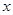= 6, 7, 10, 12, 13, 4, 8, 12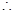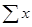= 6 + 7 + 10 + 12 + 13 + 4 + 8 + 12 = 72

And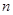= 8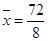= 9

Also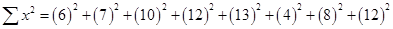= 722Variance =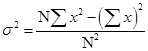=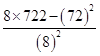=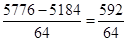= 9.25

2. Firstnatural numbers

Ans. Given: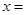1, 2, 3, 4, ………….,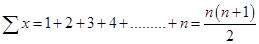And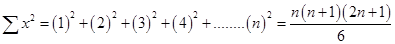Mean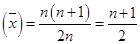Now, Variance ==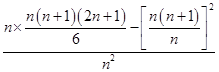=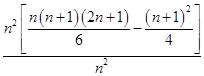=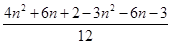=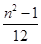3. First 10 multiples of 3

Ans. Given:3, 6, 9, 12, 15, 18, 21, 24, 27, 30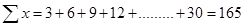And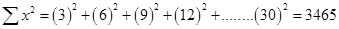Mean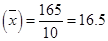Now, Variance ==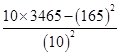=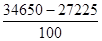=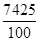= 74.25

4.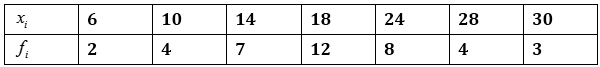Ans.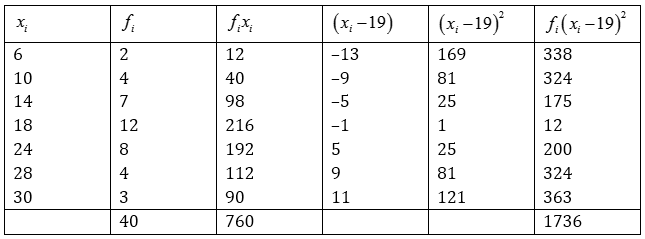Mean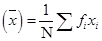=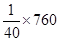= 19

Variance =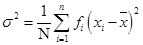=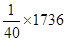= 43.4

5.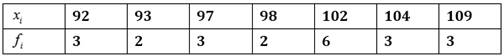Ans.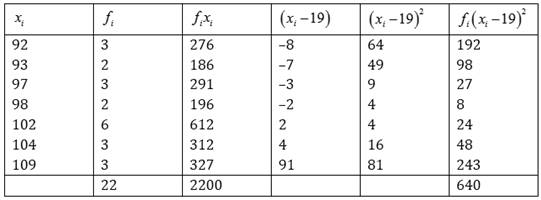Mean=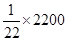= 22

Variance ==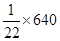= 29.09

6. Find the mean and standard deviation using short cut method.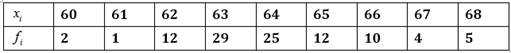Ans.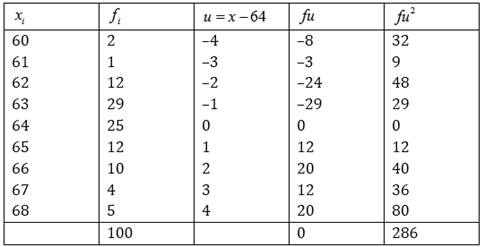Mean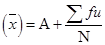=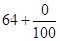= 64

Variance =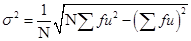=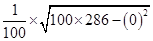=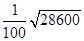=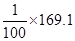= 1.69

Find the mean and variance for the following frequency distribution in Exercises 7 and 8.

7.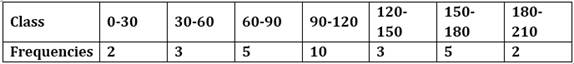Ans.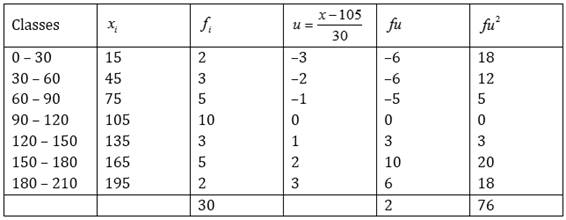Mean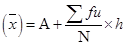=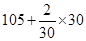= 107

Variance =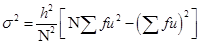=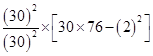= 2280 – 4 = 2276

8.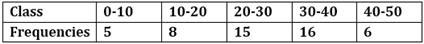Ans.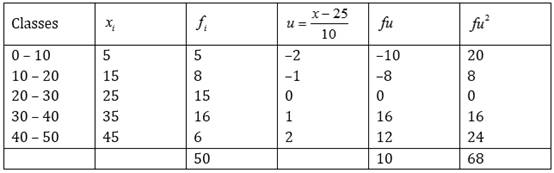Mean=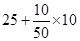= 25 + 2 = 27

Variance ==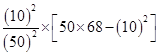=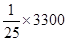= 132

9. Find the mean, variance and standard deviation using short-cut method.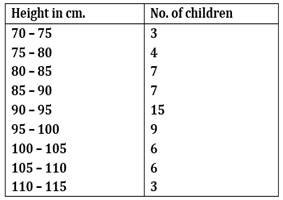Ans.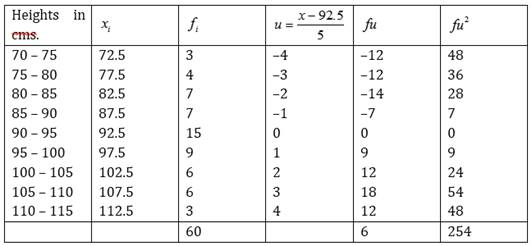Mean=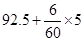= 92.5 + 0.5 = 93

Variance ==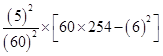=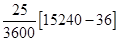=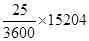= 105.58

Standard deviation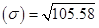= 10.27

10. The diameters of circles (in mm) drawn in a design are given below: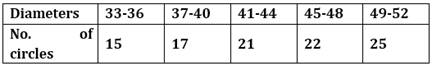Calculate the standard deviation and mean diameter of the circles.

[Hint: First make the data continuous by making the classes as 32.5-36.5, 36.5-40.5, 40.5-44.5, 44.5-48.5, 48.5-52.5 and the proceed]

Ans.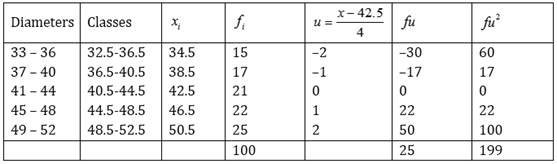Mean=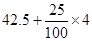= 42.5 + 1 = 43.5 mm

Standard deviation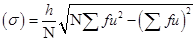=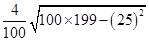=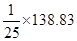= 5.55### 3 thoughts on “NCERT Solutions class-11 Maths Exercise 15.2”

1. thanks sir

2. Thanks for giving such accurate and easy solution.